# GUESSFM Plotting

Chris Wallace // web // email

# Some example data

``````mydir <-  system.file("extdata",package="GUESSFM")
``````
``````##  "X"    "Y"    "tags" "d"    "dx"   "sm"   "sp"
``````

# Prior/posterior number of SNPs in the model

`pp.nsnp` calculates a posterior distribution for the number of causal SNPs in the region, and with an argument `plot=TRUE` will also plot the distribution. If you supply the prior expected number of causal SNPs, you can compare the posterior to the prior.

``````pp.nsnp(d,plot=TRUE)
``````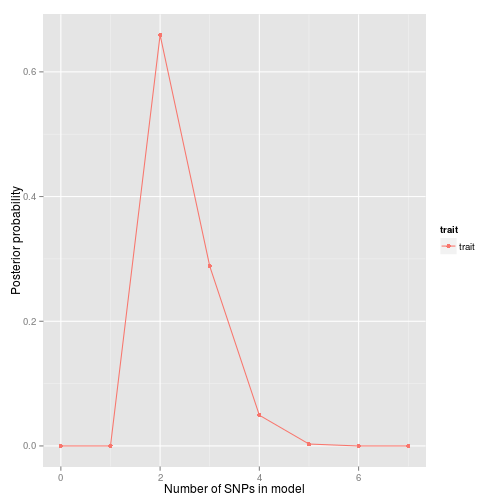``````## \$trait
##            0            1            2            3            4
## 6.352132e-46 2.209605e-10 6.593048e-01 2.883969e-01 4.923457e-02
##            5            6            7
## 3.049965e-03 1.367187e-05 4.553833e-08
``````
``````pp.nsnp(d,plot=TRUE,expected=3)
``````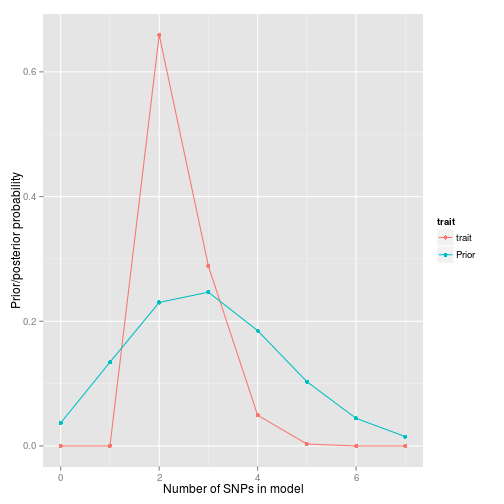``````## \$trait
##            0            1            2            3            4
## 6.352132e-46 2.209605e-10 6.593048e-01 2.883969e-01 4.923457e-02
##            5            6            7
## 3.049965e-03 1.367187e-05 4.553833e-08
``````

So that's quite nice. We had a vague-ish prior, spreading the prior weight across 0-7 SNP models, with an expected number of causal variants set at 3. We simulated data with two causal variants, and the posterior is peaked on 2 SNPs, with some weight on 3-4 SNPs.

# SNP groups

In the introduction vignette, we formed a `snppicker` object, `sp`. We can extract the SNP groups from this and use it to generate a useful summary object

``````groups <- as(sp,"groups")
groups
``````
``````## groups object, containing 5 SNPs in 2 groups.
``````
``````library(snpStats)
data(for.exercise, package="snpStats") # for SNP information
summx <- guess.summ(sm,groups=groups,snps=snp.support,position="position")
summx <- scalepos(summx,position="position")

library(ggplot2)
signals <- signal.plot(summx)
signals
``````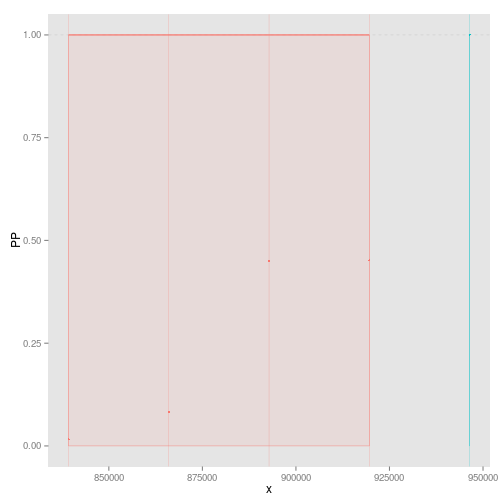``````snps <- ggsnp(summx)
snps
``````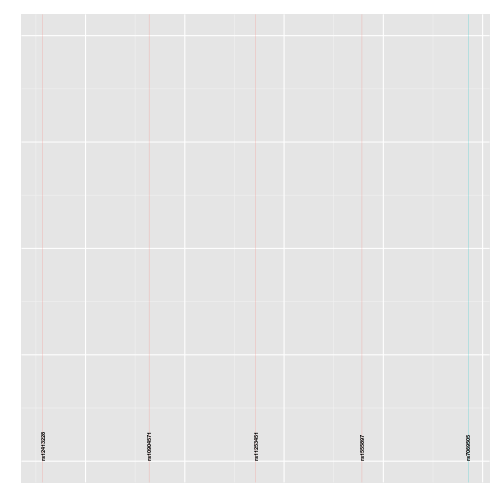``````lds <- ggld(X, summx)
lds
``````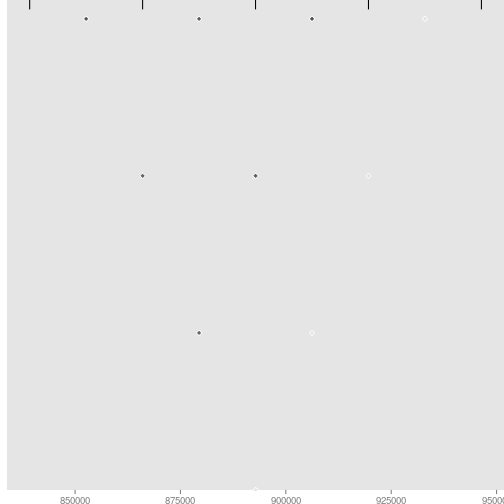``````chr <- ggchr(summx)
chr
``````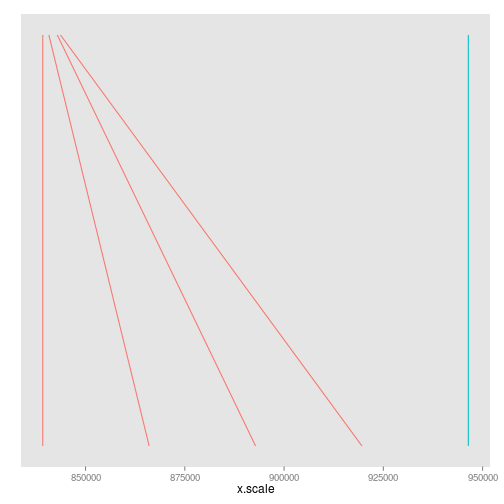Individually, perhaps only the signals plot is useful, which shows us two groups of SNPs which together have joint gMPPI close to 1. The red one contains four SNPs, and the blue group 1. The other tracks are useful to put this into some context. `snps` labels the SNPs, `lds` plots the r2 between pairs of SNPs. Grouped SNPs are ordered so SNPs in the same group are together, but this is unlikley to reflect chromosome position. `chr` is a plot of linking the group position to the chromosome position.

If you have `ggbio` installed, then this function will line up the above plots

``````library(ggbio)
tracks(chr,signals,snps,lds,heights=c(1,3,1,2))
``````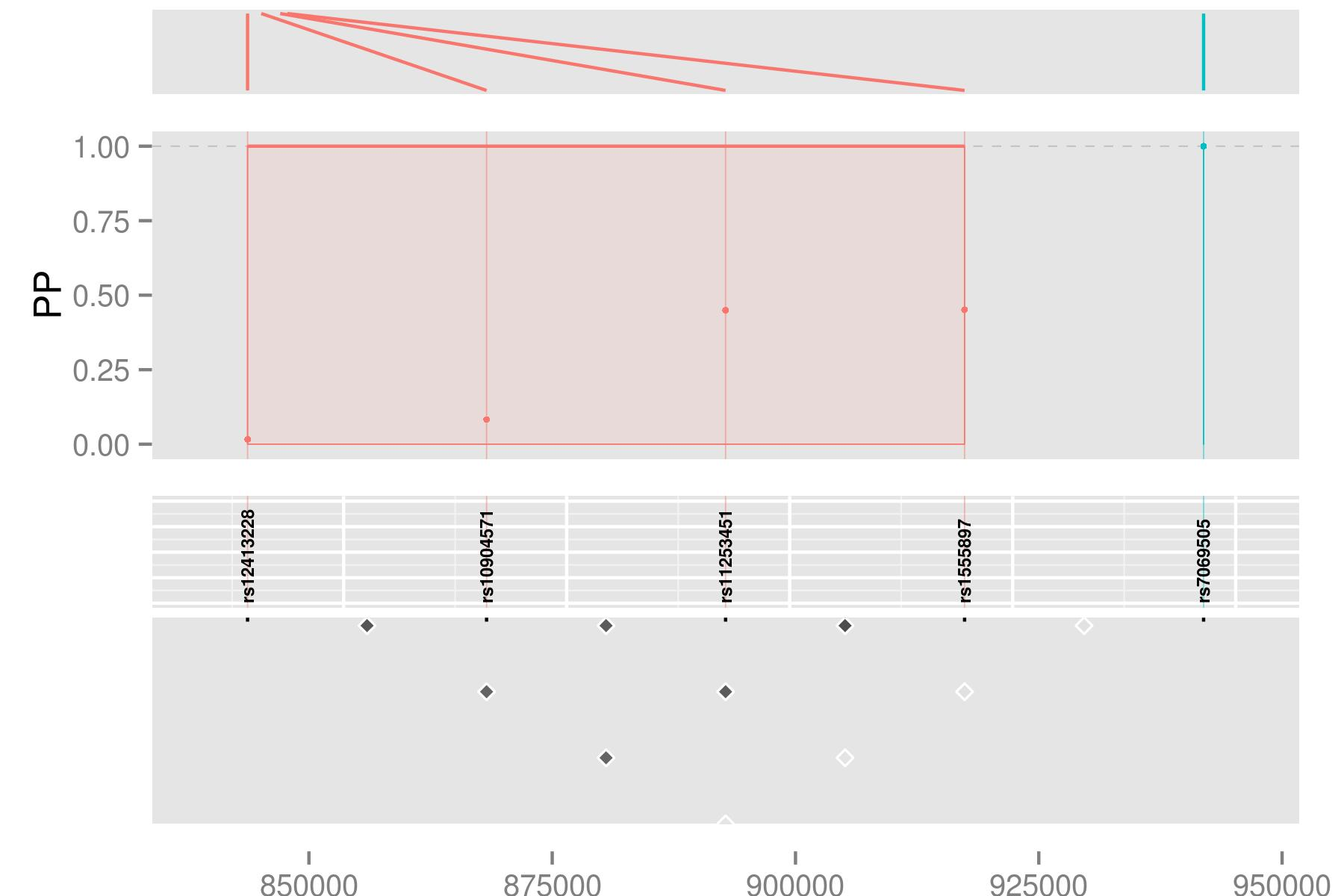This sort of thing can be useful if you have other tracks (eg, functional information) you want to line up.